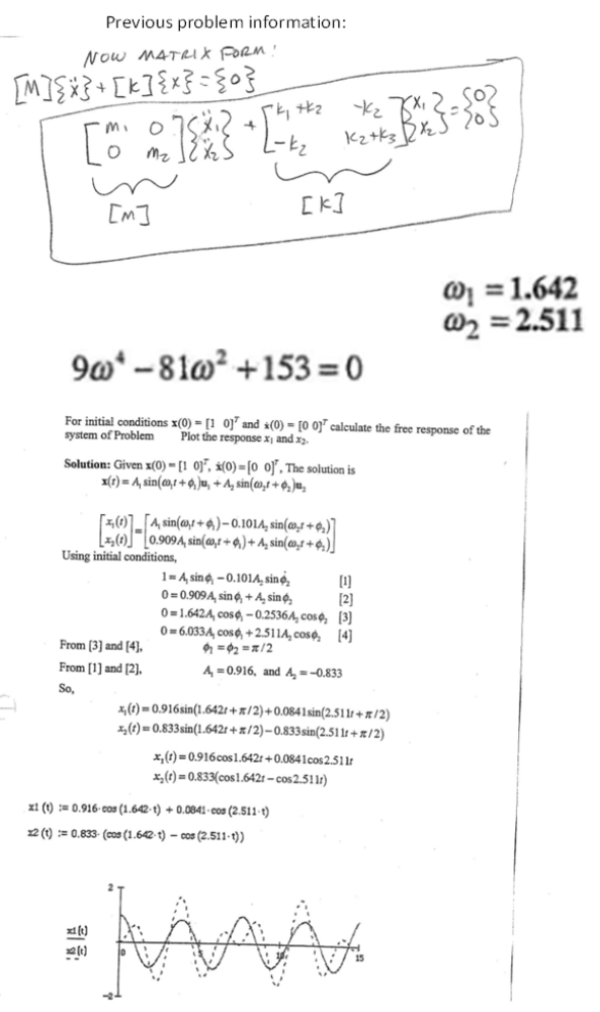For initial conditions x(0)=[1 0]^7 and xdot(0)=[0 0]^7 calculate the free response of the system of the previous problem. Plot the response x1 and x2.For initial conditions x(0)=[1 0]^7 and xdot(0)=[0 0]^7 calculate the free response of the system of the previous problem. Plot the response x1 and x2.

Vibrations Page 1 vibrations vibrations vibrations vibrations vibrations vibrations vibrations Vibrations Page 2